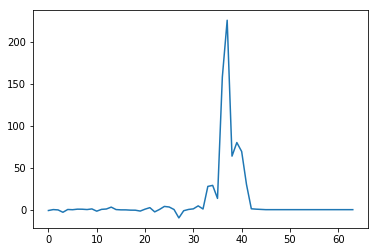# Custom loss function falling towards lowal minimum + vanishing grad

Hi!

I am working on a segmentation problem and wanted to try custom loss functions (namely dice and a variant of BCE). The functions seem to work fine as of the forward pass, but when doing backward they converge very fast to a local minimum which consists in zeroing the output mask. When I check the gradients, they tend to be very high to begin with, and then get stuck to 0 or nan when the local minima is reached. Gradient clipping doesn’t help (it is just solwer to get to the minimum). It may be specific to my problem but I believe there is something I don’t get in pytorch’s implementation, as a simple BCE doesn’t provoke this issue, while my custom version does. Below is the code for my functions (they are of course then called through a `nn.Module`):

``````def bce_loss(input, target, reduction='mean', beta=None, eps=1e-12):
n = input.size(0)
iflat = torch.sigmoid(input).view(n, -1).clamp(eps, 1-eps)
tflat = target.view(n, -1)
if not beta:
beta = 0.5
bce = -2*(beta*tflat*iflat.log()+(1-beta)*(1-tflat)*(1-iflat).log()).mean(-1)
if reduction == 'mean':
return bce.mean()
elif reduction == 'sum':
return bce.sum()
else:
return bce

def dice(input, target, smooth=1., reduction='mean'):
assert input.shape==target.shape, "input and target must have same shape"
iflat = torch.sigmoid(input).view(input.size(0), -1)
tflat = target.view(target.size(0), -1)
intersection = (iflat * tflat).sum(-1)
dice = 1 - (2. * intersection + smooth)/(iflat.sum(-1) + tflat.sum(-1) + smooth)
if reduction=='mean':
return dice.mean()
elif reduction=='sum':
return dice.sum()
else:
return dice
``````

I use mean reduction for everything. Even when I try using bce with `beta=0.5` (which is standard bce in theory), it doesn’t work. Is there any magic in autograd that I don’t get ? Do I need to define my custom backward to avoid this ?

Thanks !

EDIT: I also used gradient accumulation which may not help, but that doesn’t change the fact that pytorch’s BCE works perfectly fine, while mine doesn’t.

Your manual implementation of the binary cross entropy loss seems to work perfectly fine.
Here is a comparison using a dummy model:

``````model = nn.Sequential(
nn.Linear(1, 1),
nn.ReLU(),
nn.Linear(1, 1)
)

x = torch.randn(10, 1)
target = torch.randint(0, 2, (10, 1)).float()
criterion = nn.BCEWithLogitsLoss()

output = model(x)

loss_pytorch = criterion(output, target)
print(loss_pytorch)
loss_pytorch.backward(retain_graph=True)

loss_manual = bce_loss(output, target)
print(loss_manual)
loss_manual.backward()
``````

If you run the code, you’ll see that both losses and the corresponding gradients are equal.

If you are summing both losses together, you might need to lower the learning rate or play around with some other hyperparameters.

After some tests my custom BCE seems to work fine, even though the grads don’t exactly evolve the same way. Mine looks a bit more shaky, but nothing to really worry about I guess. However, when it comes to dice loss, even when I use it alone, it is a total catastrophy:As you see, after 30 batches I see gradients exploding then totally collapsing to 0. After 60 batches they just become `nan` (there are more than 500 batches to process in theory). Maybe I should implement a backward on this one as it looks like it is not numerically stable. It is pretty annoying because my segmentation task involves small masks, therefore dice would be quite ideal to use combined with bce. Problem is I have not yet found where this problem comes from, as I am far from an expert in pytorch’s autograd behavior. It is also possible my optimizer is causing this mess.

EDIT: looks like a smaller learning rate is indeed helping. I still don’t know why I get nans though.

The dice implementation looks fine and a sanity check yields reasonable loss values:

``````target = torch.zeros(5, 100, 100)
target[:, 50:, 50:] = 1.

for idx in range(0, 51):
input = torch.zeros(5, 100, 100)
input[:, idx:(idx+50), idx:(idx+50)] = 1.

loss = dice(input, target, reduction='mean')
print(loss)
``````

Here I just push a rectangle of ones into the target.
Note that I’ve removed the `sigmoid` call in your dice loss implementation, as I already use probabilities for `input`.
Are you dealing with a multi-class classification use case?

If the gradient is exploding, you might push the parameters into really high values, which might thus yield a nan loss.

Which optimizer are you using?

The loss doesn’t yield nan values indeed and seems to work fine for the forward part (it used to, but it was due to the edge case where probability is 0, which is problematic for the log), only the gradients do. They explode, then converge to 0 very fast and become nan. It seems like it finds a sharp local minimum where gradient can’t be computed anymore.
I’m working on semantic segmentation with only one class and a background, and I am using Adam for optimizing. I read that it can sometimes fall into local minima.

EDIT: Just as I wrote that, a training I was doing started outputting nan for the loss.

Oh I think I get it! When using dice, one pixel difference can make the loss go straight from 0 to 1. For instance, if the target is full background (so only zeros) and my prediction yields all zeros but one pixel is 1, then loss will be 1. However, changing that to full zero will directly put the loss at 0, which of course causes a very large gradient as it is totally nonlinear. That is in theory what the `smooth` variable is for, but it doesn’t seem to prevent it. I think I should try a larger value, this may very well help.

1 Like